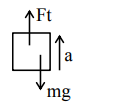# The initial mass of a rocket is

Question:

The initial mass of a rocket is $1000 \mathrm{~kg}$. Calculate at what rate the fuel should be burnt so that the rocket is given an acceleration of $20 \mathrm{~ms}^{-2}$. The gases come out at a relative speed of $500 \mathrm{~ms}^{-1}$ with respect to the rocket :[Use $g=10 \mathrm{~m} / \mathrm{s}^{2}$ ]

1. $6.0 \times 10^{2} \mathrm{~kg} \mathrm{~s}^{-1}$

2. $500 \mathrm{~kg} \mathrm{~s}^{-1}$

3. $10 \mathrm{~kg} \mathrm{~s}^{-1}$

4. $60 \mathrm{~kg} \mathrm{~s}^{-1}$

Correct Option: , 4

Solution:$\mathrm{F}_{\text {thrust }}=\left(\frac{\mathrm{dm}}{\mathrm{dt}} \cdot \mathrm{V}_{\mathrm{rel}}\right)$

$\left(\frac{\mathrm{dm}}{\mathrm{dt}} \mathrm{V}_{\mathrm{rel}}-\mathrm{mg}\right)=\mathrm{ma}$

$\Rightarrow\left(\frac{\mathrm{dm}}{\mathrm{dt}}\right) \times 500-10^{3} \times 10=10^{3} \times 20$

$\frac{\mathrm{dm}}{\mathrm{dt}}=(60 \mathrm{~kg} / \mathrm{s})$

Option (4)